xOrdered pairEncyclopedia
In mathematics
Mathematics
Mathematics is the study of quantity, space, structure, and change. Mathematicians seek out patterns and formulate new conjectures. Mathematicians resolve the truth or falsity of conjectures by mathematical proofs, which are arguments sufficient to convince other mathematicians of their validity...

, an ordered pair (a, b) is a pair of mathematical object
Mathematical object
In mathematics and the philosophy of mathematics, a mathematical object is an abstract object arising in mathematics.Commonly encountered mathematical objects include numbers, permutations, partitions, matrices, sets, functions, and relations...

s. In the ordered pair (a, b), the object a is called the first entry, and the object b the second entry of the pair. Alternatively, the objects are called the first and second coordinates, or the left and right projections of the ordered pair.

The order in which the objects appear in the pair is significant: the ordered pair (a, b) is different from the ordered pair (b, a) unless a = b.

Ordered pairs are also called 2-tuples
Tuple
In mathematics and computer science, a tuple is an ordered list of elements. In set theory, an n-tuple is a sequence of n elements, where n is a positive integer. There is also one 0-tuple, an empty sequence. An n-tuple is defined inductively using the construction of an ordered pair...

, 2-dimensional vectors
Vector (mathematics and physics)
In mathematics and physics, a vector is an element of a vector space. If n is a non negative integer and K is either the field of the real numbers or the field of the complex number, then K^n is naturally endowed with a structure of vector space, where K^n is the set of the ordered sequences of n...

, or sequence
Sequence
In mathematics, a sequence is an ordered list of objects . Like a set, it contains members , and the number of terms is called the length of the sequence. Unlike a set, order matters, and exactly the same elements can appear multiple times at different positions in the sequence...

s of length 2. The entries of an ordered pair can be other ordered pairs, enabling the recursive definition of ordered n-tuples (ordered lists of n objects). For example, the ordered triple (a,b,c) can be defined as (a, (b,c)), i.e., as one pair nested in another.

Cartesian product
Cartesian product
In mathematics, a Cartesian product is a construction to build a new set out of a number of given sets. Each member of the Cartesian product corresponds to the selection of one element each in every one of those sets...

s and binary relations (and hence the ubiquitous functions
Function (mathematics)
In mathematics, a function associates one quantity, the argument of the function, also known as the input, with another quantity, the value of the function, also known as the output. A function assigns exactly one output to each input. The argument and the value may be real numbers, but they can...

) are defined in terms of ordered pairs.

## Generalities

Let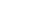and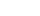be ordered pairs. Then the characteristic (or defining) property of the ordered pair is: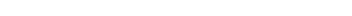The set of all ordered pairs whose first entry is in some set X and whose second entry is in some set Y is called the Cartesian product
Cartesian product
In mathematics, a Cartesian product is a construction to build a new set out of a number of given sets. Each member of the Cartesian product corresponds to the selection of one element each in every one of those sets...

of X and Y, and written X×Y. A binary relation
Binary relation
In mathematics, a binary relation on a set A is a collection of ordered pairs of elements of A. In other words, it is a subset of the Cartesian product A2 = . More generally, a binary relation between two sets A and B is a subset of...

between sets X and Y is a subset
Subset
In mathematics, especially in set theory, a set A is a subset of a set B if A is "contained" inside B. A and B may coincide. The relationship of one set being a subset of another is called inclusion or sometimes containment...

of X×Y.

If one wishes to employ the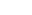notation for a different purpose (such as denoting open intervals on the real number line) the ordered pair may be denoted by the variant notation## Defining the ordered pair using set theory

The above characteristic property of ordered pairs is all that is required to understand the role of ordered pairs in mathematics. Hence the ordered pair can be taken as a primitive notion
Primitive notion
In mathematics, logic, and formal systems, a primitive notion is an undefined concept. In particular, a primitive notion is not defined in terms of previously defined concepts, but is only motivated informally, usually by an appeal to intuition and everyday experience. In an axiomatic theory or...

, whose associated axiom is the characteristic property. This was the approach taken by the N. Bourbaki group in its Theory of Sets, published in 1954, long after Kuratowski discovered his reduction (below). The Kuratowski definition was added in the second edition of Theory of Sets, published in 1970.

If one agrees that set theory
Set theory
Set theory is the branch of mathematics that studies sets, which are collections of objects. Although any type of object can be collected into a set, set theory is applied most often to objects that are relevant to mathematics...

is an appealing foundation of mathematics
Foundations of mathematics
Foundations of mathematics is a term sometimes used for certain fields of mathematics, such as mathematical logic, axiomatic set theory, proof theory, model theory, type theory and recursion theory...

, then all mathematical objects must be defined as sets of some sort. Hence if the ordered pair is not taken as primitive, it must be defined as a set. Several set-theoretic definitions of the ordered pair are given below.

### Wiener's definition

Norbert Wiener
Norbert Wiener
Norbert Wiener was an American mathematician.A famous child prodigy, Wiener later became an early researcher in stochastic and noise processes, contributing work relevant to electronic engineering, electronic communication, and control systems.Wiener is regarded as the originator of cybernetics, a...

proposed the first set theoretical definition of the ordered pair in 1914 :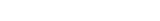He observed that this definition made it possible to define the types
Type theory
In mathematics, logic and computer science, type theory is any of several formal systems that can serve as alternatives to naive set theory, or the study of such formalisms in general...

of Principia Mathematica
Principia Mathematica
The Principia Mathematica is a three-volume work on the foundations of mathematics, written by Alfred North Whitehead and Bertrand Russell and published in 1910, 1912, and 1913...

as sets. Principia Mathematica had taken types, and hence relations
Relation (mathematics)
In set theory and logic, a relation is a property that assigns truth values to k-tuples of individuals. Typically, the property describes a possible connection between the components of a k-tuple...

of all arities, as primitive
Primitive notion
In mathematics, logic, and formal systems, a primitive notion is an undefined concept. In particular, a primitive notion is not defined in terms of previously defined concepts, but is only motivated informally, usually by an appeal to intuition and everyday experience. In an axiomatic theory or...

.

Wiener used instead of {b} to make the definition compatible with Type Theory
Type theory
In mathematics, logic and computer science, type theory is any of several formal systems that can serve as alternatives to naive set theory, or the study of such formalisms in general...

where all elements in a class must be of the same "type". With nesting b within an additional set its type is made equal to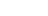's.

### Hausdorff's definition

About the same time as Wiener (1914), Felix Hausdorff
Felix Hausdorff
Felix Hausdorff was a Jewish German mathematician who is considered to be one of the founders of modern topology and who contributed significantly to set theory, descriptive set theory, measure theory, function theory, and functional analysis.-Life:Hausdorff studied at the University of Leipzig,...

proposed his definition: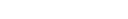"where 1 and 2 are two distinct objects different from a and b" .

### Kuratowski definition

In 1921 Kuratowski offered the now-accepted definition of the ordered pair (a, b):Note that this definition is used even when the first and the second coordinates are identical:Given some ordered pair p, the property "x is the first coordinate of p" can be formulated as: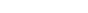The property "x is the second coordinate of p" can be formulated as: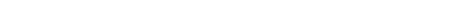In the case that the left and right coordinates are identical, the right conjunct
Conjunct
In linguistics, the term conjunct has three distinct uses:*A conjunct is an adjunct that adds information to the sentence that is not considered part of the propositional content but which connects the sentence with previous parts of the discourse...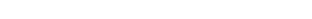is trivially true, since Y1Y2 is never the case.

This is how we can extract the first coordinate of a pair (using the notation for arbitrary intersection and arbitrary union):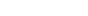This is how the second coordinate can be extracted: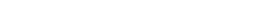#### Variants

The above Kuratowski definition of the ordered pair is "adequate" in that it satisfies the characteristic property that an ordered pair must satisfy, namely that. There are other definitions, of similar or lesser complexity, that are equally adequate:
•••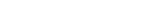reverse is merely a trivial variant of the Kuratowski definition, and as such is of no further interest. short is so-called because it requires two rather than three pairs of braces
Bracket
Brackets are tall punctuation marks used in matched pairs within text, to set apart or interject other text. In the United States, "bracket" usually refers specifically to the "square" or "box" type.-List of types:...

. Proving that short satisfies the characteristic property requires the Zermelo–Fraenkel set theory
Zermelo–Fraenkel set theory
In mathematics, Zermelo–Fraenkel set theory with the axiom of choice, named after mathematicians Ernst Zermelo and Abraham Fraenkel and commonly abbreviated ZFC, is one of several axiomatic systems that were proposed in the early twentieth century to formulate a theory of sets without the paradoxes...

axiom of regularity
Axiom of regularity
In mathematics, the axiom of regularity is one of the axioms of Zermelo-Fraenkel set theory and was introduced by...

Moreover, if one accepts the standard set-theoretic construction of the natural numbers, then 2 is defined as the set {0, 1} = {0, {0}}, which is indistinguishable from the pair (0, 0)short. Yet another disadvantage of the short pair is the fact, that even if a and b are of the same type, the elements of the short pair are not.

#### Proving that definitions satisfy the characteristic property

Prove: (a, b) = (c, d) if and only if
If and only if
In logic and related fields such as mathematics and philosophy, if and only if is a biconditional logical connective between statements....

a = c and b = d.

Kuratowski:

If. If a = c and b = d, then = . Thus (a, b)K = (c, d)K.

Only if. Two cases: a = b, and ab.

If a = b:K = = = .K = = .
Thus {c} = {c, d} = {a}, which implies a = c and a = d. By hypothesis, a = b. Hence b = d.

If ab, then (a, b)K = (c, d)K implies = .
Suppose {c, d} = {a}. Then c = d = a, and so = = = . But then would also equal , so that b = a which contradicts ab.

Suppose {c} = {a, b}. Then a = b = c, which also contradicts ab.

Therefore {c} = {a}, so that c = a and {c, d} = {a, b}.

If d = a were true, then {c, d} = {a, a} = {a} ≠ {a, b}, a contradiction. Thus d = b is the case, so that a = c and b = d.

Reverse:

(a, b)reverse = = = (b, a)K.

If. If (a, b)reverse = (c, d)reverse,
(b, a)K = (d, c)K. Therefore b = d and a = c.

Only if. If a = c and b = d, then = .
Thus (a, b)reverse = (c, d)reverse.

Short:

If: Obvious.

Only if: Suppose {a, {a, b}} = {c, {c, d}}.
Then a is in the left hand side, and thus in the right hand side.
Because equal sets have equal elements, one of a = c or a = {c, d} must be the case.
If a = {c, d}, then by similar reasoning as above, {a, b} is in the right hand side, so {a, b} = c or {a, b} = {c, d}.
If {a, b} = c then c is in {c, d} = a and a is in c, and this combination contradicts the axiom of regularity, as {a, c} has no minimal element under the relation "element of."
If {a, b} = {c, d}, then a is an element of a, from a = {c, d} = {a, b}, again contradicting regularity.
Hence a = c must hold.

Again, we see that {a, b} = c or {a, b} = {c, d}.
The option {a, b} = c and a = c implies that c is an element of c, contradicting regularity.
So we have a = c and {a, b} = {c, d}, and so: {b} = {a, b} \ {a} = {c, d} \ {c} = {d}, so b = d.

### Quine-Rosser definition

Rosser (1953) employed a definition of the ordered pair, due to Quine
Willard Van Orman Quine
Willard Van Orman Quine was an American philosopher and logician in the analytic tradition...

and requiring a prior definition of the natural number
Natural number
In mathematics, the natural numbers are the ordinary whole numbers used for counting and ordering . These purposes are related to the linguistic notions of cardinal and ordinal numbers, respectively...

s. Let be the set of natural numbers, and define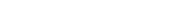Applying this function simply increments every natural number in x. In particular,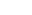does not contain the number 0, so that for any sets x and y,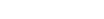Define the ordered pair (A, B) as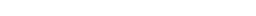Extracting all the elements of the pair that do not contain 0 and undoing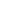yields A. Likewise, B can be recovered from the elements of the pair that do contain 0.

In type theory
Type theory
In mathematics, logic and computer science, type theory is any of several formal systems that can serve as alternatives to naive set theory, or the study of such formalisms in general...

and in outgrowths thereof such as the axiomatic set theory NF
New Foundations
In mathematical logic, New Foundations is an axiomatic set theory, conceived by Willard Van Orman Quine as a simplification of the theory of types of Principia Mathematica. Quine first proposed NF in a 1937 article titled "New Foundations for Mathematical Logic"; hence the name...

, the Quine-Rosser pair has the same type as its projections and hence is termed a "type-level" ordered pair. Hence this definition has the advantage of enabling a function
Function (mathematics)
In mathematics, a function associates one quantity, the argument of the function, also known as the input, with another quantity, the value of the function, also known as the output. A function assigns exactly one output to each input. The argument and the value may be real numbers, but they can...

, defined as a set of ordered pairs, to have a type only 1 higher than the type of its arguments. This definition works only if the set of natural numbers is infinite. This is the case in NF
New Foundations
In mathematical logic, New Foundations is an axiomatic set theory, conceived by Willard Van Orman Quine as a simplification of the theory of types of Principia Mathematica. Quine first proposed NF in a 1937 article titled "New Foundations for Mathematical Logic"; hence the name...

, but not in type theory
Type theory
In mathematics, logic and computer science, type theory is any of several formal systems that can serve as alternatives to naive set theory, or the study of such formalisms in general...

or in NFU
New Foundations
In mathematical logic, New Foundations is an axiomatic set theory, conceived by Willard Van Orman Quine as a simplification of the theory of types of Principia Mathematica. Quine first proposed NF in a 1937 article titled "New Foundations for Mathematical Logic"; hence the name...

. J. Barkley Rosser showed that the existence of such a type-level ordered pair (or even a "type-raising by 1" ordered pair) implies the axiom of infinity
Axiom of infinity
In axiomatic set theory and the branches of logic, mathematics, and computer science that use it, the axiom of infinity is one of the axioms of Zermelo-Fraenkel set theory...

. For an extensive discussion of the ordered pair in the context of Quinian set theories, see Holmes (1998).

### Morse definition

Morse-Kelley set theory (Morse 1965) makes free use of proper classes. Morse defined the ordered pair so that its projections could be proper classes as well as sets. (The Kuratowski definition does not allow this.) He first defined ordered pairs whose projections are sets in Kuratowski's manner. He then redefined the pair (x, y) as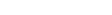, where the component Cartesian products are Kuratowski pairs on sets. This second step renders possible pairs whose projections are proper classes. The Quine-Rosser definition above also admits proper classes as projections.

## Category theory

A category-theoretic product
Product (category theory)
In category theory, the product of two objects in a category is a notion designed to capture the essence behind constructions in other areas of mathematics such as the cartesian product of sets, the direct product of groups, the direct product of rings and the product of topological spaces...

A x B in a category of sets
Category of sets
In the mathematical field of category theory, the category of sets, denoted as Set, is the category whose objects are sets. The arrows or morphisms between sets A and B are all functions from A to B...

represents the set of ordered pairs, with the first element coming from A and the second coming from B. In this context the characteristic property above is a consequence of the universal property
Universal property
In various branches of mathematics, a useful construction is often viewed as the “most efficient solution” to a certain problem. The definition of a universal property uses the language of category theory to make this notion precise and to study it abstractly.This article gives a general treatment...

of the product and the fact that elements of a set X can be identified with morphisms from 1 (a one element set) to X. While different objects may have the universal property, they are all naturally isomorphic.